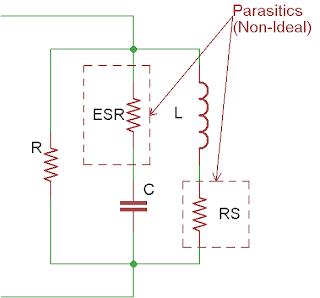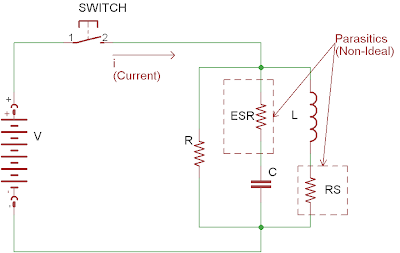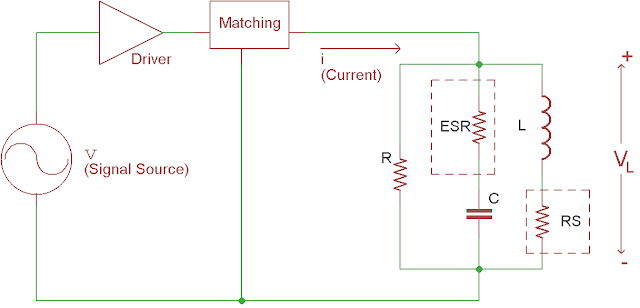This blog has permanently moved tohttps://boseji.com

Thank you for all your support.

Saturday, September 22, 2012

Inductive Coupling De-simplified: NFC Antenna

For a long time we wondered how does inductive coupling works. It not until recently that we had a revelations:
```Φ = v / ω
Where,
v – AC Voltage applied to the Coil
[volt = Joules/Coulomb = kg.m²/(Ampere . second³)]
Φ - Magnetic Flux(Instantaneous)
[Weber = Joule . Second / Coulomb = Joule / Ampere =
kg.m²/(Ampere . second²)]
```

This is a very important relationship that shows how magnetic coupling really behaves. Taking it from this result we have the following conclusions:
1. As the Angular Frequency decreases (ω = 2.π.freq) Flux increases
2. As AC voltage applied increases then Flux increases
Hence this principle can be used for a wide variety of applications such as:
• RF-ID Antenna
• Inductive Charging
• NFC
Well we will now tell you how we came to this equation finally. Thanks to Mr. Francois Pichon for explaining us in such intricate details of this phenomenon.

To start our discussion we keep in context the NFC antenna. Lately, we were trying to use the SeeedStudio's NFC Shield, we wanted to adapt this shield to become a complete NFC reader. However we wanted to make a board of our own. This presented us with a challenge to implement the Antenna as well as understand the working of the Magnetic coupling.

Beginning with the Basics here is the Basic Antenna that we can look at.Figure 1
This is a basically an inductor to begin with. But to receive the signal or to be able to send out signal we need to generate magnetic field and that too with the specified frequency. This is done by making a Tank Circuit for that specified frequency. These are circuits whose effective Impedance nulls out or maximizes at the Resonance Frequency. Basically we need to add a capacitor to make it L C Parallel Tuned Circuit.

We can choose the appropriate value from the formula:
```L . C = ω´²
Where,
L - Self Inductance of the Antenna [Henry]
ω´ - Resonant Angular Frequency [radians/second]
```

Thus, the New Circuit we have is this:Figure 2
We also know that these components used in the circuit are not always ideal. Especially the Inductor has a loss and the capacitor has ESR. Howsoever small this would shift our intended ω´ from the one that we had designed. Thus we add one more compensating resistor in parallel to this. Thus effective circuit with the paracitics and compensation.Figure 3
Now the value of this resistor depends on the individual components. It cant be determined directly however while doing measurements depending on the frequency deviation this can determined by replacement technique.

So, now we have a circuit whose properties are dependent on the Frequency of the signal that we provide to excite this circuit.

Let us now try to see how to power this circuit from switched source.Figure 4
This above figure shows the method of driving the circuit using an DC voltage source and a switch. The operation of the switch is to turn on and turn off the power to the coil this creating the time varying signal to excite the circuit.
There are some important points to note about this circuit. The Inductor is a Current impeding element; means Current through the Inductor cant change instantaneously and Voltage across the Inductor can change instantaneously.
If VL is the voltage across the inductor and i is the current through it then we have.
```VL= L . di / dt   --(Eq1)
Where,
L - Inductance [Henry]
i - Current Through Inductor [Ampere]
t - Time [Seconds]
```

This means that there is a possibility of the Inductor dumping back energy while the Switch is turned off in the above circuit.
Now towards the Explanation of the Magnetic flux developed by the inductor. We know from the magnetic field basics and Lenz's Law that Magnetic flux (Φ) is proportional to the current flowing though the conductor.
``` That is,
Φ = L . I   --(Eq2)
Where,
L - Inductance of the Conductor [Henry]
I - Instantaneous DC Current [Ampere]
Φ - Instantaneous Magnetic Flux Developed [Weber]
```

Now to simplify let us consider the current supplied to the Inductor is AC and similarly for the Voltage also.
```Hence,
vl = L . di / dt  --(Eq3) [from Eq1]
Also, i = i cos(ωθ) [AC Signal with 90° Phase Shift due to Inductor]

So We have applying into Eq3:

vl = L . ω . i´  --(Eq4)
Where,
i´ - Phase Shifted AC Current [Ampere]
```

Now taking into account the Instantaneous behavior of the Eq2 and by substituting with Eq4 we obtain the Grand Relation.
```Hence,
Φ = L . vl / ( L . ω )
[From Eq4 substituting (i Ξ i´) ]
We have,
Φ = vl / ω
```

The interesting part of this explanation is that the finally the Flux Φ is independent of the Inductance L and the AC Current i. Thus, in order to have the maximum flux linkage and energy transfer, we need to maximize the Voltage vl if Frequency ω needs to be constant.

Still the question remains how to maximize the vl and also supress the energy dumping issue due to nature of the Inductor. As in partical circuit there might not be any isolating swtich. It might only be a simple Transistor driver that runs the coil.

The answer to this is what is called as Matching.
We can use capacitive isolation to minimize the energy dumping and diode on the transmitting end to reduce the energy dumping.And to maximize the voltage further we need to use a Push-Pull Driver rnning at higher voltage to maximize the voltage transition observed across the Inductor.

The Chip used in the Seeed Studio NFC Shield is the PN532 device from NXP Semiconductors. There are some good application notes that help in antenna design for this device. Here are a list of the links that can help you design:
We would publish our final design calculation on this chip. So that you too can design your own NFC boards.Figure 5